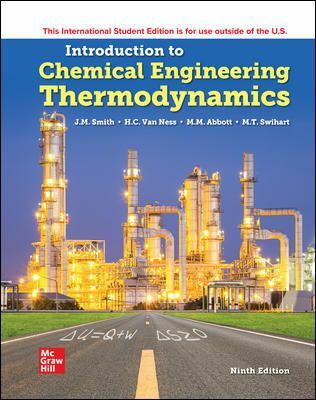•# Introduction to Chemical Engineering Thermodynamics, 9th Edition

RM 97.00
- +

9th Edition

Introduction to Chemical Engineering Thermodynamics presents comprehensive coverage of thermodynamics from a chemical engineering viewpoint. The text provides a thorough exposition of the principles of thermodynamics and details their application to chemical processes. The chapters are written in a clear, logically organized manner, and contain an abundance of realistic problems, examples, and illustrations to help students understand complex concepts. This text is structured to alternate between the development of thermodynamic principles and the correlation and use of thermodynamic properties as well as between theory and applications.

1) Introduction2) The First Law and Other Basic Concepts
3) Volumetric Properties of Pure Fluids
4) Heat Effects
5) The Second Law of Thermodynamics
6) Thermodynamic Properties of Fluids
7) Applications of Thermodynamics to Flow Processes
8) Production of Power from Heat
9) Refrigeration and Liquefaction
10) The Framework of Solution Thermodynamics
11) Mixing Processes
12) Phase Equilibrium: Introduction
13) Thermodynamic Formulations for Vapor/Liquid Equilibrium
14) Chemical-Reaction Equilibria
15) Topics in Phase Equilibria
16) Thermodynamic Analysis of Processes